# Python | Pandas Index.intersection()

• Last Updated : 17 Dec, 2018

Python is a great language for doing data analysis, primarily because of the fantastic ecosystem of data-centric python packages. Pandas is one of those packages and makes importing and analyzing data much easier.

Pandas` Index.intersection()` function form the intersection of two Index objects. This returns a new Index with elements common to the index and other, preserving the order of the calling index.

Syntax: Index.intersection(other)

Parameters :
other : Index or array-like

Returns : intersection : Index

Example #1: Use `Index.intersection()` function to find the set intersection of two Indexes.

 `# importing pandas as pd``import` `pandas as pd`` ` `# Creating the first Index``idx1 ``=` `pd.Index([``'Labrador'``, ``'Beagle'``, ``'Mastiff'``, ``                     ``'Lhasa'``, ``'Husky'``, ``'Beagle'``])`` ` `# Creating the second Index``idx2 ``=` `pd.Index([``'Labrador'``, ``'Great_Dane'``, ``'Pug'``,``           ``'German_sepherd'``, ``'Husky'``, ``'Pitbull'``])`` ` `# Print the first and second Index``print``(idx1, ``'\n'``, idx2)`

Output :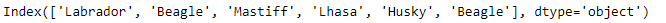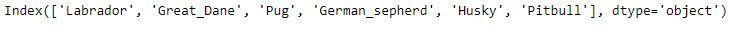Now we find the set intersection of the two Indexes.

 `# Find the elements common to both the Indexes``idx2.intersection(idx1)`

Output :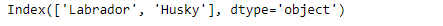As we can see in the output, the `Index.intersection()` function has returned the intersection of the two indexes. The ordering of the labels has been maintained based on the calling Index.

Example #2: Use `Index.intersection()` function to find the set intersection of two Indexes. The Index contains `NaN` values.

 `# importing pandas as pd``import` `pandas as pd`` ` `# Creating the first Index``idx1 ``=` `pd.Index([``'2015-10-31'``, ``'2015-12-02'``, ``None``, ``'2016-01-03'``, ``                      ``'2016-02-08'``, ``'2017-05-05'``, ``'2014-02-11'``])`` ` `# Creating the second Index``idx2 ``=` `pd.Index([``'2015-10-31'``, ``'2015-10-02'``, ``'2018-01-03'``,``           ``'2016-02-08'``, ``'2017-06-05'``, ``'2014-07-11'``, ``None``])`` ` `# Print the first and second Index``print``(idx1, ``'\n'``, idx2)`

Output :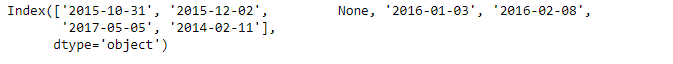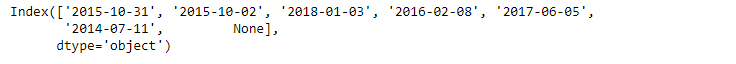Now we find the intersection of idx1 and idx2.

 `# find intersection and maintain ``# ordering of labels based on idx1``idx1.intersection(idx2)`

Output :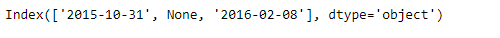Note : The missing values in both the indexes are considered common to each other.

My Personal Notes arrow_drop_up